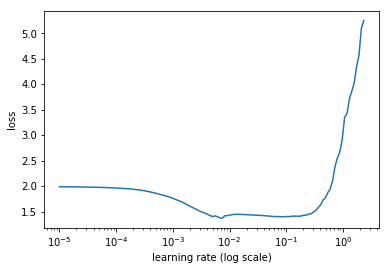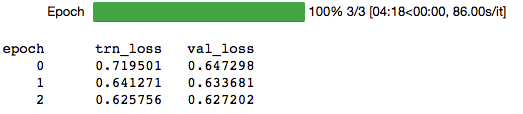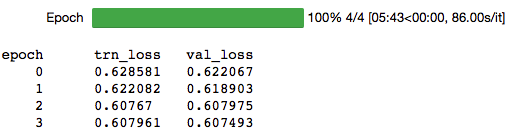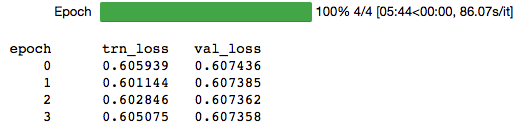# Autoencoder for gene sequences

Hi,

in one of my recent projects I’m trying to build a autoencoder to encode long gene sequences in a smaller latent vector to search sequences and try to find similarities between different sequences. Since the Sequences are quite long (up to >60.000) I used a combination of conv layers and LSTM units. For a proof of concept I limited the data to sequences with a length of <= 3000. The dataset I used can be found here.
As loss function I’m using cross_entropy and the loss is decreasing within the first epochs, however I cant get it any lower than 0.6 on train and validation data. And the reconstruction from the latent vector does not look any close to the input:

``````print(val_df.seq.values)
``````

ATGTGTCCCCGAGCCGCGCGGGCGCCCGCGACGCTACTCCTCGCCCTGGGCGCGGTGCTGTGGCCTGCGGCTGGCGCCTGGGAGCTTACGATTTTGCACACCAACGACGTGCACAGCCGGCTGGAGCAGACCAGCGAGGACTCCAGCAAGTGCGTCAACGCCAGCCGCTGCATGGGTGGCGTGGCTCGGCTCTTCACCAAGGTTCAGCAGATCCGCCGCGCCGAACCCAACGTGCTGCTGCTGGACGCCGGCGACCAGTACCAGGGCACTATCTGGTTCACCGTGTACAAGGGCGCCGAGGTGGCGCACTTCATGAACGCCCTGCGCTACGATGCCATGGCACTGGGAAATCATGAATTTGATAATGGTGTGGAAGGACTGATCGAGCCACTCCTCAAAGAGGCCAAATTTCCAATTCTGAGTGCAAACATTAAAGCAAAGGGGCCACTAGCATCTCAAATATCAGGACTTTATTTGCCATATAAAGTTCTTCCTGTTGGTGATGAAGTTGTGGGAATCGTTGGATACACTTCCAAAGAAACCCCTTTTCTCTCAAATCCAGGGACAAATTTAGTGTTTGAAGATGAAATCACTGCATTACAACCTGAAGTAGATAAGTTAAAAACTCTAAATGTGAACAAAATTATTGCACTGGGACATTCGGGTTTTGAAATGGATAAACTCATCGCTCAGAAAGTGAGGGGTGTGGACGTCGTGGTGGGAGGACACTCCAACAC…

``````latent = ae.encode(x=val_df.ids.values)
ae.sample(latent)
``````

ATGGAGGGGGGGGGGGGGGGGGGGGGGGGCCTGGCCGGGGGGGGGGCCCCCCCCCCCCCCCCCCCCCCCCCCCCCCCCCCCCCCCCCCCCCCCCCCCCCCCCCCCCCCCCCCCCCCCCCCCCCCCCCCCCCCCCCCCCCCCCCCCCCCCCCCCCCCCCCCCCCCCCCCCCCCCCCCCCCCCTGGCCCTGGCCCTGGAGGAGGAGGAGGAGGAGGAGGAGGAGGAGGAGGAGGAGGAGGAGGAGGAGGAGGAGGAGGAGGAGGAGGAGGAGGAGGAGGAGGAGGAGGAGGAGGAGGAGGAGGAGGAGGAGGAGGAGGAGGAGGAGGAGGAGGAGGAGGAGGAGGAGGAGGAGGAGGAGGAGGAGGAGGAGGAGGAGGAGGAGGAAGAGGAGGAGGAGGAGGAGGAGGAGGAGGAGGAGGAGGAGGAGGAGGAGGAGGAGGAGGAGGAAGAAGAAGAAGAAGAAGAAGAAGAAGAAGAAGAAGAAGAAGAAGAAGAAGAAGAAGAAGAAGAAGAAGAAGAAGAAGAAGAAGAAGAAGAAGAAGAAGAAGAAGAAGAAGAAGAAGAAAAAAAAAAAAAAAAAGAAGAAGAAAAAGAAGAAGAAGAAGAAGAAGAAGAAGAAGAAGAAGAAGAAGAAGAAGAAGAAGAAGAAGAAGAAGAAGAAGAAGAAGAAGAAAAAGAAAAAAAAAAAAAAAAAAAAAAAAAAAAAGAAGAAGAAGAAGAAGAAGAAAAAAAAAAAGAAAAAAAAAAAAAAAAAAAAAAAAAAAAAAAAAAAAAAAAAAAAAAAAAAAAAAAAAAAAAAAAAAAAAAAAAAAAAAAAAAAAAAAAAAAAAAAAAAAAAAAAAAAAAAAAAAAAAAAAAAAAAAAAAAAAAAAAAAAAAAAAAAAAAAAAAAAAAAAAAAAAAAAAAAAAAAAAAAAAAAAAAAAAAAAAAAAAAAAAAAAAAAAAAAAAAAAAAAAAAAAAAAAAAAAAAAAAAAAAAAAAAAAAAAAAAAAAAAAAAAAAAAAAAAAAAAAAAAAAAAAAAAAGAAAAAAAAAAAAAAAAAAAAAAAAAAAAAAAAAAAAAAAAAAAAAAAAAAAAAAAAAAAAAAAAAAAAAAAAAAAAAAAAAAAAAAAAAAAAAAAGAAAAAAAAAAAAAAAAAAAAAAAAAAAAAAAAAAAGAAGAAGAAGAAGAAGAAGAAGAAGAAGAAGAAGAAGAAGAAGAAGAAGAAGAAGAAGAAGAAGAAGAAGAAG…

Are there any suggestions on improving the encoding? This is the model I’m currently using:

``````class AutoEncoder(nn.Module):
def __init__(self,vocab_size, embed_size, latent_size,filters,decoder_out_filters,
stoi,itos,GO_TOKEN,STOP_TOKEN):
super().__init__()

self.latent_size = latent_size
self.vocab_size = vocab_size
self.embed_size = embed_size
self.seqLength = seqLength
self.itos = itos
self.stoi = stoi
self.GO = GO_TOKEN
self.STOP = STOP_TOKEN
self.decoder_out_filters = decoder_out_filters

self.encFilters = filters.copy()
filters.reverse()
self.decFilters = filters.copy()

self.embed = nn.Embedding(self.vocab_size, self.embed_size)
self.embed.weight = xavier_normal_(self.embed.weight)

self.encoder = Encoder(embed_size,latent_size,self.encFilters)
self.decoder = Decoder(vocab_size,latent_size,50,2,embed_size,self.decFilters,out_filters=decoder_out_filters)

def forward(self,encIn,decIn):
encIn = self.embed(encIn)
encOut = self.encoder(encIn)

decIn = self.embed(decIn)
decOut = self.decoder(encOut,decIn)

return decOut

def encode(self,x):

if isinstance(x,list):
x = np.asarray(x)[None]

res = np.zeros((len(x), self.seqLength), dtype=x.dtype) + self.pad_idx
for i,o in enumerate(x):
if          self.pre_pad: res[i, -len(o):] = o
else:       res[i,  :len(o)] = o

emb = self.embed(V(T(res)))
latent = self.encoder(emb)
return latent

def sample(self,latent):
final_state = None

x = self.embed(V(T([self.stoi[self.GO]])[None]))

result = [self.GO]

cnn_out = self.decoder.conv_decoder(latent)

for var in t.transpose(cnn_out,0,1):
out, final_state = self.decoder.rnn_decoder(var.unsqueeze(1),decoder_input=x,initial_state=final_state)
out = F.softmax(out.squeeze())
out = to_np(out)

idx = out.argmax()
char = self.itos[idx]
result.append(char)

if char == self.STOP:
break

x = self.embed(V(T([idx])[None]))

return ''.join(result)
``````

Encoder:

``````class ConvBlock(nn.Module):
def __init__(self,prev,nf,kernelsize,stride):
super().__init__()
self.convblock = nn.Sequential(nn.Conv1d(prev, nf, kernelsize, stride),
nn.BatchNorm1d(nf),
nn.ELU())

def forward(self,x):
return self.convblock(x)

class Encoder(nn.Module):
def __init__(self, embed_size, latent_size,filters):
super().__init__()

self.embed_size = embed_size
self.latent_size = latent_size

filters = [self.embed_size]+filters+[self.latent_size]
convblocks = []
for i in range(1,len(filters)):
if i == 9:
kernel = 2
else:
kernel = 4
convblocks.append(ConvBlock(filters[i-1],filters[i],kernel,2))

self.cnn = nn.Sequential(*convblocks)

def forward(self,x):
x = t.transpose(x, 1, 2)
result = self.cnn(x)
result = result.squeeze(2)

return result
``````

Decoder:

``````class DeconvBlock(nn.Module):
super().__init__()
self.convblock = nn.Sequential(nn.ConvTranspose1d(prev, nf, kernelsize, stride,0, padding),
nn.BatchNorm1d(nf),
nn.ELU())

def forward(self,x):
return self.convblock(x)

class Decoder(nn.Module):
def __init__(self,vocab_size, latent_variable_size, rnn_size, rnn_num_layers, embed_size, filters,out_filters):
super().__init__()
self.vocab_size = vocab_size
self.latent_variable_size = latent_variable_size
self.rnn_size = rnn_size
self.embed_size = embed_size
self.rnn_num_layers = rnn_num_layers
self.out_filters = out_filters

filters = [self.latent_variable_size]+filters+[self.out_filters]#+[self.vocab_size]
convblocks = []
for i in range(1,len(filters)):
kernel = 4
if i == 2:
kernel = 2

if i in [2,3,5,6,7,9]:

self.cnn = nn.Sequential(*convblocks)

self.rnn = nn.GRU(input_size=self.out_filters + self.embed_size,
hidden_size=self.rnn_size,
num_layers=self.rnn_num_layers,
batch_first=True)

self.hidden_to_vocab = nn.Linear(self.rnn_size, self.vocab_size)

def forward(self,latent_variable,decoder_input):
aux_logits = self.conv_decoder(latent_variable)

logits, _ = self.rnn_decoder(aux_logits, decoder_input, initial_state=None)

return logits

def rnn_decoder(self, cnn_out, decoder_input, initial_state=None):
logits, final_state = self.rnn(t.cat([cnn_out, decoder_input], 2), initial_state)

[batch_size, seq_len, _] = logits.size()
logits = logits.contiguous().view(-1, self.rnn_size)

logits = self.hidden_to_vocab(logits)

logits = logits.view(batch_size, seq_len, self.vocab_size)

return logits, final_state

def conv_decoder(self, latent_variable):
latent_variable = latent_variable.unsqueeze(2)

out = self.cnn(latent_variable)
out = t.transpose(out, 1, 2).contiguous()
return out
``````

LR-Find:
I tried several parameter settings, which all led to a loss around 0.6-ish.

``````layers = [128,128,128,256,256,256,512,512,512]
ae = AutoEncoder(len(itos),200,512,layers,
decoder_out_filters = 512,
seqLength=3000,
GO_TOKEN=GO,
STOP_TOKEN=STOP,
itos=itos,
stoi=stoi)
learn = RNN_Learner(md, SingleModel(to_gpu(ae)))
learn.crit = celoss
````````````lr = 1e-3
learn.fit(lr,1,cycle_len=3,cycle_mult=3,use_wd_sched=False,use_clr=(20,10))
````````````lr = 1e-4
learn.fit(lr,1,cycle_len=4,cycle_mult=2)
````````````lr = 1e-6
learn.fit(lr,1,cycle_len=4,cycle_mult=2)
``````2 Likes

Hello.
How does the convolution layer help you to encode the gene sequences,
and search for the similarities?

I thought CNN were used for image recognition?
Are you just going to show the CNN a picture of the dataset gene sequences??

My intuition is that you probably want to look at encoding a vocabulary of n-grams. A number of languages like chinese don’t include separators so there’s been work to figure out how to combine. Even 3000 characters is a lot to expect an LSTM to remember. If you could take that sequence and turn it into a few hundred n-grams, especially if they repeat in patterns you’d probably have better luck.

@bladerunner2945 There’s a lot of work on CNNs for text. If you’re interested in NLP I’d suggest checking out CS231n from stanford available on youtube as it covers a lot of different NLP methods.

Even

Auto-encoder for Gene Sequences".

Did you use a CNN along with an Auto encoder algorithm??
Because I see a “class DeconvBlock(nn.Module)” you are using,
Is this part of a CNN??

@bladerunner2945 maybe I skipped some of the important stuff. I’m not using image data. I used textdata, my gene sequences consist of 4 capital letters (A, T, G, C) plus a STOP, GO and Padding token. With these I build a vocabulary of length 7 and feed this into an embedding layer in the beginning.
Actually I copied and adapted the implemantation of kefirski (https://github.com/kefirski/hybrid_rvae) which is a implementation of the “A Hybrid Convolutional Variational Autoencoder for Text Generation” Paper (https://arxiv.org/abs/1702.02390). I just skipped the variational part for now and was going to implement it in the next version to see how much it improves the results. But if my understanding is right, it just helps to prevent overfitting.
As Even already mentioned 3000 characters (or even more if you think about the sequences which are over 60.000 characters) are a lot to ask for, I used convolutional / deconv layers to reduce the sequence length.

With the conv layers I’m reducing dimensions from [-1,3000,embedding] to [-1,number_of_filters,1] which then gets fed into the LSTM and finally in a linear layer to get the latent vector. So the LSTM will never get the full sequence.

I will definitely try the ngrams next.
Nevertheless, I’m wondering if my dataset is too small? I’m using just 4525 observations. Would it help if I could get more data?

1 Like

Ok, so just to re-iterate…
you CAN use Convolutional Variational Autoencoders to
generate new text data information based on inputs??

The reason why I am asking is
I am designing a GAN to design new chemical molecules
and I am wondering which type of GAN I should use??

Why did you start with a vocabulary length of 7?? Is this
7 characters you mean???

Yes, you can use conv layers followed by a LSTM to generate Text Sequences.

Yes the vocabulary is 7 characters, 4 Characters for the gene sequences (“A”, “T”, “G” & “C”) + 3 additional Tokens: one for the beginning of the gene sequence, one to mark the end of a sequence and a special token for padding. My stoi-Dict look like this:

`defaultdict(int, {'': 0, '<': 1, '>': 2, 'G': 3, 'A': 4, 'T': 5, 'C': 6})`

I tried using n-grams now and also a pure RNN Autoencoder, still no luck. It always gets stuck at a certain point of loss. Any other ideas?

Do you only need the encodings or are you also interested in the VAE approach?

If it’s only the (DNA) encoding this could be interesting for you:

I’m very interested in this topic but so far I didn’t used deep learning for biotech data.

Best regards
Michael

The following paper might be worth looking into for generative models of DNA sequences: Generating and designing DNA with deep generative models

Do you have a ground-truth of similar sequence pairs? If yes, you could directly train a siamese model on that.

Side note: one problem with convolutional autoencoders is that they can easily learn the identity (discussed in 'Winner-Take-All autoencoders paper)

2 Likes

Thank you for the information - I have to look through it!Thanks for the resources. They look pretty interesting.

I mostly need the encodings, but I’m interested in VAE as well. Anyhow I would prefer my own trained model, since I want to include other descriptive features for gene sequences later.

If I understood GAN’s right, they are not really what I am looking for. In my understanding GAN’s sample from a random latent vector to generate new Sequences. I would like to do it the other way around, I have given sequences which i want to embed in a latent vector.

Sadly I do not have any similar paris of sequences, just the sequences it self. I have no background on bioinformatics or gene sequences, so there might be a way to detect similarities.

I did some testing with my models, I created a toy-dataset which is a random sequence of given characters. I’m trying 3 different kinds of VAE now, one version with only dense/fully-connected layers, a RNN version and a combination von Conv-layers and RNN. All versions are able to embed a sequence of 2 characters into a vector of length 1 with a loss of close to 0. - Yay

However, if I increase sequence length to say 10 and a vocab size of 4 characters, the loss get stuck at these numbers:

• latent vector size = 2 --> loss = 0.9
• latent vector size = 5 --> loss = 0.5
• latent vector size = 7 --> loss = 0.3
• latent vector size = 10 --> loss = 0.05

I do get the feeling, that my implementation seems to be correct and these losses are kind of “global” minima. I’m just wondering how other papers did a better job. I.e. this Paper:

embeds sequences of 3288 into a 2-d latent vector with a reconstruction loss of 0.2. With “just” one hot encoding, while I am also using embedding for the characters. When I’m using a sequence of 3000 and an latent vector of 2 using their architecture of [1600, 800, 400, 150, 2] neurons my loss plateaus at about 1.38.